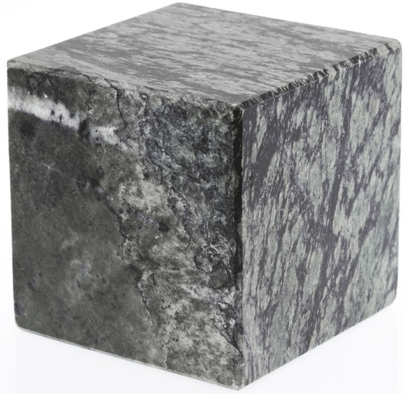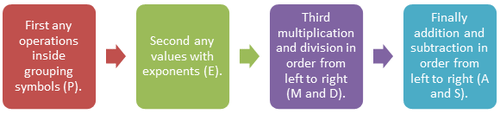# 1.4.5: Order of Operations and Variable Substitution

•• Contributed by CK12
• CK12

## Evaluate Polynomial ExpressionsFigure $$\PageIndex{1}$$

Mr. Travis is taking his Social Studies class on a tour of downtown. He has created a scavenger hunt for the students as they travel around the city. The scavenger hunt is made up of all different types of architecture and landmarks as well as problems that will need to be solved. Mr. Travis asked the bus to drop the students off in front of the Town Hall. In the square across from the town hall is a plaza with three cubes in it.

One of the problems on the sheet read “Before you is a cube. Use the formula A=6s2 to find the surface area of a cube whose side measures 8 feet.”

Can you solve this problem?

In this concept, you will learn to evaluate polynomial expressions.

### Evaluating Polynomials

A polynomial is an algebraic expression that shows the sum of monomials.

In order to solve algebraic expressions, you need to use the order of operations, commonly called PEMDAS. The diagram below shows the order of arithmetic operations performed using PEMDAS.Figure $$\PageIndex{1}$$

When you consider expressions, you can evaluate an expression for a given value. In other words, you can find the total value if you know the value that corresponds to the variable. You can replace the variable(s) with the given value and then use the order of operations to calculate the total value.

Let’s look at an example.

Evaluate x2+3x−10 for x=5

First, replace the variables with the given value of x.

(5)2+3(5)−10

Next, find the total value using the order of operations.

(5)2+3(5)−10

25+15−10 Complete the multiplication.

40−10 Perform the addition and subtraction from left to right.

30

### Examples

Example $$\PageIndex{1}$$

Earlier, you were given a problem about the cube. You have been asked to find the surface area of a cube that has a side length of 8 feet.

Solution

First, substitute the side length into the formula for the surface area.

A=6s2

A=6(8)2

Next, use PEMDAS to solve for the surface area.

A=6(8)2 First evaluate the exponent 82=8×8=64.

A=6(64) Perform the multiplication.

A=384

The surface area of the cube is 384 ft2.

Example $$\PageIndex{1}$$

Evaluate 4x2+2x+15 for x=3

Solution

First, replace the variables with the given value of x.

4(3)2+2(3)+15

Next, find the total value using the order of operations.

4(3)2+2(3)+15

36+6+15 Complete the multiplication.

42+15 Perform the addition from left to right.

57

Example $$\PageIndex{1}$$

Evaluate x2+5x−1 for x=3

Solution

First, replace the variables with the given value of x.

(3)2+5(3)−1

Next, find the total value using the order of operations.

(3)2+5(3)−1

9+15−1 Complete the multiplication.

24−1 Perform the addition and subtraction from left to right.

23

Example $$\PageIndex{1}$$

Evaluate x2+4x−9 for x=2

Solution

First, replace the variables with the given value of x.

(2)2+4(2)−9

Next, find the total value using the order of operations.

(2)2+4(2)−9

4+8−9 Complete the multiplication.

12−9 Perform the addition and subtraction from left to right.

3

Example $$\PageIndex{1}$$

Evaluate 2x2+2x+5 for x=3

Solution

First, replace the variables with the given value of x.

2(3)2+2(3)+5

Next, find the total value using the order of operations.

(3)2+2(3)+5

18+6+5 Complete the multiplication.

24+5 Addition from left to right.

29

### Review

Evaluate the following expressions for the given value.

1. 7x3 for x=2
2. 6x2 for x=3
3. 4x3 for x=2
4. 8x2 for x=2
5. 10xy for x=2,y=3
6. 7x2+4x for x=2
7. 6x2+5x for x=2
8. 3x2+8x for x=3
9. 7x2+4x−2 for x=2
10. 9x2+5x−3 for x=3
11. 5x2+5x−2 for x=2
12. 12x2+8x+11 for x=2
13. 6y2−2y−8 for y−6
14. 3(x−7)+5(x+1) for x=10
15. −2y3+6(y−4)+y for y=−3

### Vocabulary

Term Definition
Binomial A binomial is an expression with two terms. The prefix 'bi' means 'two'.
Coefficient A coefficient is the number in front of a variable.
constant A constant is a value that does not change. In Algebra, this is a number such as 3, 12, 342, etc., as opposed to a variable such as x, y or a.
like terms Terms are considered like terms if they are composed of the same variables with the same exponents on each variable.
Monomial A monomial is an expression made up of only one term.
Polynomial A polynomial is an expression with at least one algebraic term, but which does not indicate division by a variable or contain variables with fractional exponents.
Trinomial A trinomial is a mathematical expression with three terms.